# Solving Laplace's equation in polar coordinates for specific boundary conditions

• A
• Stefan H

#### Stefan H

TL;DR Summary
Solving Laplace's equation for magnetic potentials inside and outside a ferromagnetic wire with specific boundary conditions. Help needed in understanding how to obtain the solution.
Hello everybody,

Currently I am doing my master's thesis and I've encountered a physics problem which is very difficult for me to solve. The problem I have is finding equations for the magnetic scalar potential inside and outside a ferromagnetic wire for specific boundary conditions.

Explanation of the problem:
A ferromagnetic wire is located in an external magnetic field. This means there is a magnetic potential inside and outside of the wire. I want to find specific solutions on the surface of the wire (r=a).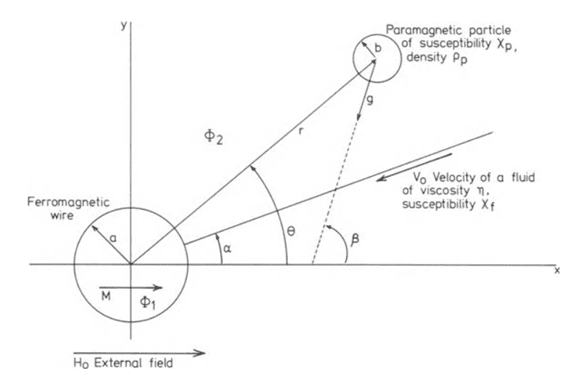My solution approach was to first solve Laplace's equation for polar coordinates for both magnetic potentials: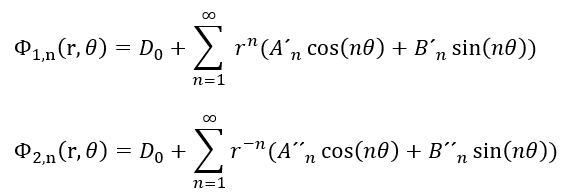Now I want to find an expression for both potentials on the surface of the wire to obtain this solution (According to Gerber: "Applied Magnetism", 1994):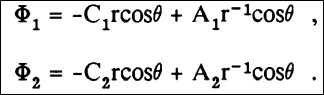with the given boundary conditions: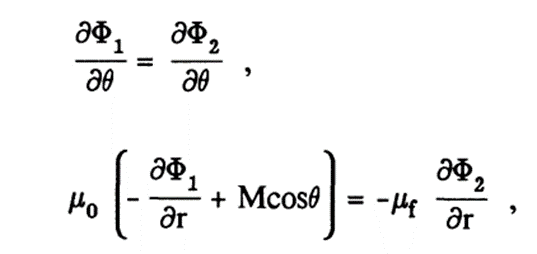The next goal would be to determine the constants C1, A1, C2 and A2. However, I really don't get how Gerber came to this type of solution. He only mentioned to use Laplace's equation and the boundary conditions with quote: "linear combination of cylindrical harmonics" to get his solution.

My overall goal is to understand his approach for this kind of problem, so I can apply it to a different geometry. It would be very nice if anybody here could help me out :)

Thanks in advance and happy Holidays,
Stefan

Last edited:

Summary:: Solving Laplace's equation for magnetic potentials inside and outside a ferromagnetic wire with specific boundary conditions. Help needed in understanding how to obtain the solution.

Hello everybody,

Currently I am doing my master's thesis and I've encountered a physics problem which is very difficult for me to solve. The problem I have is finding equations for the magnetic scalar potential inside and outside a ferromagnetic wire for specific boundary conditions.

Explanation of the problem:
A ferromagnetic wire is located in an external magnetic field. This means there is a magnetic potential inside and outside of the wire. I want to find specific solutions on the surface of the wire (r=a).
View attachment 294600

My solution approach was to first solve Laplace's equation for polar coordinates for both magnetic potentials:View attachment 294601

Now I want to find an expression for both potentials on the surface of the wire to obtain this solution (According to Gerber: "Applied Magnetism", 1994):

View attachment 294602

with the given boundary conditions:

View attachment 294603

The next goal would be to determine the constants C1, A1, C2 and A2. However, I really don't get how Gerber came to this type of solution. He only mentioned to use Laplace's equation and the boundary conditions with quote: "linear combination of cylindrical harmonics" to get his solution.

My overall goal is to understand his approach for this kind of problem, so I can apply it to a different geometry. It would be very nice if anybody here could help me out :)

Thanks in advance and happy Holidays,
Stefan

The method is to obtain the Fourier series for $\partial \Phi/\partial r$ on the boundary, and determine the coefficients of $r^{\pm n}\cos(n\theta)$ and $r^{\pm n}\sin(n\theta$ accordingly. In this case that is straightforward: the Fourier series for $\mu_0M\cos\theta$ is $\mu_0M\cos\theta$. Thus from the boundary conditions you get the following simultaneous equations for $n \geq 1$: $$\begin{split} A_n' - A_n'' &= 0\\ B_n' - B_n'' &= 0\\ \mu_0A_n' + \mu_fA_n'' &= \begin{cases} \mu_0M, & n = 1 \\ 0 & n > 1, \end{cases} \\ \mu_0B_n' + \mu_fB_n'' &= 0 \end{split}$$ These have the unique solution $A_n' = A_n'' = 0$ for $n >1$ while $A_1' = A_1''$ is non-zero, with $B_n' = B_n'' = 0$ for all $n$.

Once you become sufficiently familiar with second-order linear ODEs, it will be intuitively obvious that only the $r^{\pm1}\cos\theta$ terms were required here.

•Stefan H

However I still have some questions left:
1. Why is it necessary to obtain the Fourier series? The way I would proceed on this problem is to form the derivative depending on theta and r separately and put it in the boundary conditions. However, this would just lead to more equations which I don't know how to simplify.
2. Maybe I put it wrong, but A´n, A´´n, B´n and B´´n are just coefficients. They don't mean the derivative of something. Is the answer still true then?
3. I sadly don't really understand why the Fourier series of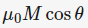is required, how it is obtained and how the following simultaneous equations are obtained. If you could elaborate on that, it would be very helpful.

The method is to obtain the Fourier series for $\partial \Phi/\partial r$ on the boundary, and determine the coefficients of $r^{\pm n}\cos(n\theta)$ and $r^{\pm n}\sin(n\theta$ accordingly. In this case that is straightforward: the Fourier series for $\mu_0M\cos\theta$ is $\mu_0M\cos\theta$. Thus from the boundary conditions you get the following simultaneous equations for $n \geq 1$: $$\begin{split} A_n' - A_n'' &= 0\\ B_n' - B_n'' &= 0\\ \mu_0A_n' + \mu_fA_n'' &= \begin{cases} \mu_0M, & n = 1 \\ 0 & n > 1, \end{cases} \\ \mu_0B_n' + \mu_fB_n'' &= 0 \end{split}$$ These have the unique solution $A_n' = A_n'' = 0$ for $n >1$ while $A_1' = A_1''$ is non-zero, with $B_n' = B_n'' = 0$ for all $n$.

Once you become sufficiently familiar with second-order linear ODEs, it will be intuitively obvious that only the $r^{\pm1}\cos\theta$ terms were required here.
Sorry that I am posting that again, but maybe you didn't see my answer since I did not reply directly to you.